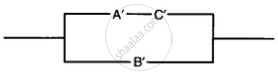# If A, B and C Are the Elements of Boolean Algebra, Simplify the Expression (A’ + B’) (A + C’) + B’ (B + C). Draw the Simplified Circuit. - Mathematics

Sum

If A, B and C are the elements of Boolean algebra, simplify the expression (A’ + B’) (A + C’) + B’ (B + C). Draw the simplified circuit.

#### Solution

(A' + B')(A + C') + B'(B + C)

= A' (A + C') + B' (A + C') + B'B + B'C

= A'A + A'C' + B'A + B'C' + B'B + B'C

= 0 + A'C' + B'A + B'C' + 0 + B'C         .....[∵  A'A = 0]

= A'C' + B'A + B' (C'+C)

= A'C'+B'A+B'                    .....[∵ A + A' = 1]

= A'C' + B' (A + 1)

= A'C' +B'                            ......[∵ A + 1 = 1]

simplified circuit :-Concept: Introduction of Relations and Functions
Is there an error in this question or solution?